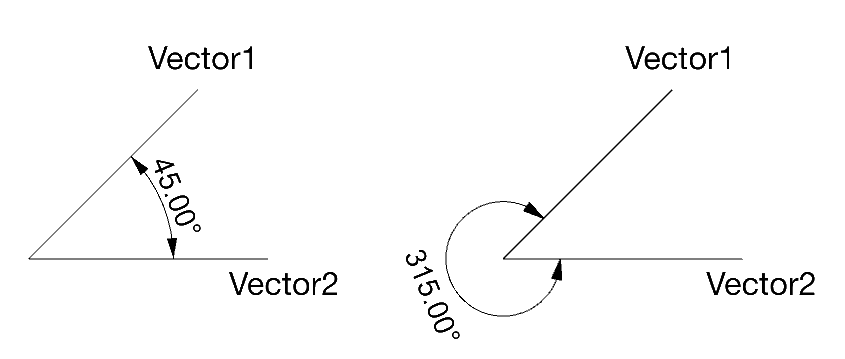Calculating the Angle Between Two Points
Windows only

## Problem

For two sets of 3D vectors, you can use the method demonstrated in Calculate the Angle Between Two Vectors to calculate the angle between them. The results for both are always the same - 45 degree in this case. For example:But what if you need a result in which one is 45 degrees the other is 315 degrees?

## Solution

The following example code demonstrates how to calculate the angle between two 3D points, given a common base point.

/*
Description:
Calculates the angle between two points that lie on a
given plane.
Parameters:
plane   - [in]  The plane on which the points lie
basept  - [in]  The base point
refpt1  - [in]  The first reference point
refpt2  - [in]  The second reference point
Returns:
TRUE if successful, FALSE otherwise.
*/
static bool CalculatePlaneAngle(
const ON_Plane& plane,
const ON_3dPoint& basept,
const ON_3dPoint& refpt1,
const ON_3dPoint& refpt2,
)
{
// Make sure the points are on the plane
double tolerance = 0.000001;
double dist = 0.0;

dist = plane.plane_equation.ValueAt( basept );
if( fabs(dist) > tolerance )
return false;

dist = plane.plane_equation.ValueAt( refpt1 );
if( fabs(dist) > tolerance )
return false;

dist = plane.plane_equation.ValueAt( refpt2 );
if( fabs(dist) > tolerance )
return false;

// Make sure base and reference points are not equal

if( basept == refpt1 | basept == refpt2 )
return false;

// Calculate angle between vectors

ON_3dVector v = refpt2 - basept;
v.Unitize();

ON_3dVector zerov = refpt1 - basept;
zerov.Unitize();

double dot = ON_DotProduct( zerov, v );
dot = RHINO_CLAMP( dot, -1.0, 1.0 );
double angle = acos( dot );

// Calculate a new y-axis based on the plane's
// zaxis and our zero vector
v = ON_CrossProduct( plane.zaxis, zerov );
v.Unitize();

// Create a plane using our y-axis a the normal
ON_Plane yplane;
yplane.CreateFromNormal( basept, v );

// Figure out which side of this plane that refpt2 is on
dist = yplane.plane_equation.ValueAt( refpt2 );
if( dist < 0.0 )
angle = (ON_PI * 2.0) - angle;

return true;
}


You can use the above function as follows…

CRhinoCommand::result CCommandTest::RunCommand( const CRhinoCommandContext& context )
{
CRhinoView* view = RhinoApp().ActiveView();
if( 0 == view )
return failure;

ON_Plane plane = view->ActiveViewport().ConstructionPlane().m_plane;
ON_3dPoint basept, refpt1, refpt2;

CRhinoGetPoint gp;
gp.SetCommandPrompt( L"Base point" );
gp.Constrain( plane );
gp.GetPoint();
if( gp.CommandResult() != success )
return gp.CommandResult();

basept = gp.Point();

gp.SetCommandPrompt( L"First angle point" );
gp.SetBasePoint( basept );
gp.DrawLineFromPoint( basept, TRUE );
gp.GetPoint();
if( gp.CommandResult() != success )
return gp.CommandResult();

refpt1 = gp.Point();

gp.SetCommandPrompt( L"Second angle point" );
gp.SetBasePoint( basept );
gp.DrawLineFromPoint( basept, TRUE );
gp.GetPoint();
if( gp.CommandResult() != success )
return gp.CommandResult();

refpt2 = gp.Point();

double angle = 0.0;
if( CalculatePlaneAngle(plane, basept, refpt1, refpt2, angle) )
RhinoApp().Print( L"Angle = %.3f degrees.\n", angle * (180.0 / ON_PI) );

return success;
}